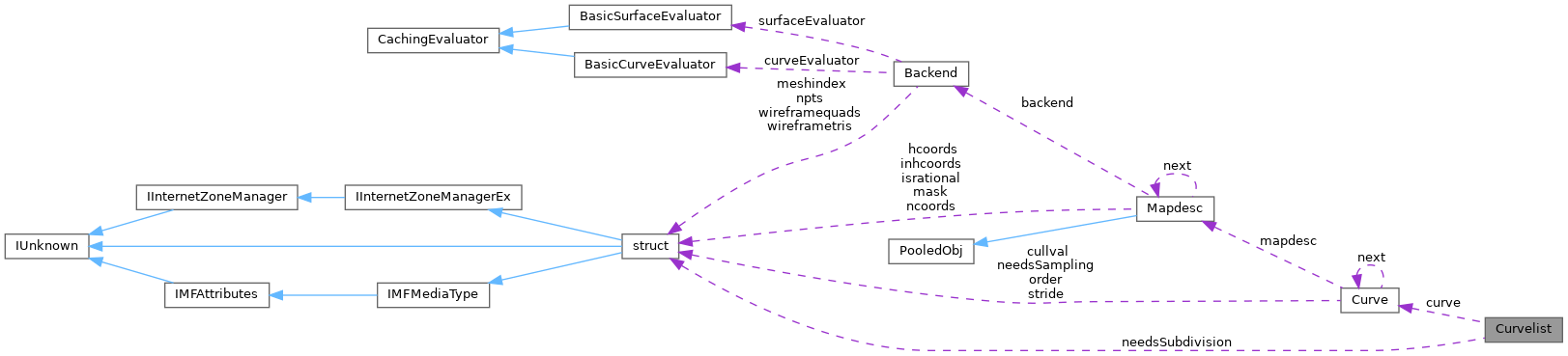ReactOS 0.4.15-dev-7196-g0fe0b40
Curvelist Class Reference

`#include <curvelist.h>`

Collaboration diagram for Curvelist:[legend]

## Public Member Functions

Curvelist (Quilt *, REAL, REAL)

Curvelist (Curvelist &, REAL)

~Curvelist (void)

int cullCheck (void)

void getstepsize (void)

int needsSamplingSubdivision ()

## Private Attributes

Curvecurve

float range 

int needsSubdivision

float stepsize

class Subdivider

## Detailed Description

Definition at line 46 of file curvelist.h.

## ◆ Curvelist() [1/2]

 Curvelist::Curvelist ( Quilt * quilts, REAL pta, REAL ptb )

Definition at line 48 of file curvelist.cc.

49{
50 curve = 0;
51 for( Quilt *q = quilts; q; q = q->next )
52 curve = new Curve( q, pta, ptb, curve );
53 range = pta;
54 range = ptb;
55 range = ptb - pta;
57 stepsize = 0;
58}
Definition: curve.h:46
float stepsize
Definition: curvelist.h:60
int needsSubdivision
Definition: curvelist.h:59
Curve * curve
Definition: curvelist.h:57
Definition: quilt.h:64
GLdouble GLdouble GLdouble GLdouble q
Definition: gl.h:2063
GLenum GLint * range
Definition: glext.h:7539

## ◆ Curvelist() [2/2]

 Curvelist::Curvelist ( Curvelist & upper, REAL value )

Definition at line 60 of file curvelist.cc.

61{
62 curve = 0;
63 for( Curve *c = upper.curve; c; c = c->next )
64 curve = new Curve( *c, value, curve );
65
66 range = upper.range;
67 range = value;
68 range = value - upper.range;
69 upper.range = value;
70 upper.range = upper.range - value;
72 stepsize = 0;
73}
Curve * next
Definition: curve.h:51
float range
Definition: curvelist.h:58
const GLubyte * c
Definition: glext.h:8905
Definition: pdh_main.c:94

## ◆ ~Curvelist()

 Curvelist::~Curvelist ( void )

Definition at line 75 of file curvelist.cc.

76{
77 while( curve ) {
78 Curve *c = curve;
79 curve = curve->next;
80 delete c;
81 }
82}
#define c
Definition: ke_i.h:80

## ◆ cullCheck()

 int Curvelist::cullCheck ( void )

Definition at line 85 of file curvelist.cc.

86{
87 for( Curve *c = curve; c; c = c->next )
88 if( c->cullCheck() == CULL_TRIVIAL_REJECT )
90 return CULL_ACCEPT;
91}
#define CULL_ACCEPT
Definition: defines.h:42
#define CULL_TRIVIAL_REJECT
Definition: defines.h:40
struct define * next
Definition: compiler.c:65

Referenced by Subdivider::samplingSplit().

## ◆ getstepsize()

 void Curvelist::getstepsize ( void )

Definition at line 94 of file curvelist.cc.

95{
96 stepsize = range;
97 Curve *c;
98 for( c = curve; c; c = c->next ) {
99 c->getstepsize();
100 c->clamp();
101 stepsize = ((c->stepsize < stepsize) ? c->stepsize : stepsize);
102 if( c->needsSamplingSubdivision() ) break;
103 }
104 needsSubdivision = ( c ) ? 1 : 0;
105}

Referenced by Subdivider::samplingSplit().

## ◆ needsSamplingSubdivision()

 int Curvelist::needsSamplingSubdivision ( void )

Definition at line 108 of file curvelist.cc.

109{
110 return needsSubdivision;
111}

Referenced by Subdivider::samplingSplit().

## ◆ Subdivider

 friend class Subdivider
friend

Definition at line 48 of file curvelist.h.

## ◆ curve

 Curve* Curvelist::curve
private

Definition at line 57 of file curvelist.h.

Referenced by cullCheck(), Curvelist(), getstepsize(), and ~Curvelist().

## ◆ needsSubdivision

 int Curvelist::needsSubdivision
private

Definition at line 59 of file curvelist.h.

Referenced by Curvelist(), getstepsize(), and needsSamplingSubdivision().

## ◆ range

 float Curvelist::range
private

Definition at line 58 of file curvelist.h.

Referenced by Curvelist(), and Subdivider::samplingSplit().

## ◆ stepsize

 float Curvelist::stepsize
private

Definition at line 60 of file curvelist.h.

Referenced by Curvelist(), getstepsize(), and Subdivider::samplingSplit().

The documentation for this class was generated from the following files: